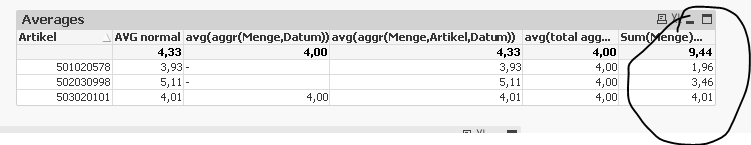# QlikView Deployment

Discussion Board for collaboration related to QlikView Deployment.

Highlighted
New Contributor III

## Avg with missing Data (aggr?)

Hello experts,

I have a Problem with calculating the right avg values:

The Problem in short is as follows - There is an article, that only consumption for 1 day in a month. let's say 01.01.2017.

In the datamodle there are only rows, where there had been consumption.

I want to calculate the average daily consumption

In that case - if I use the avg function the formula will return 1, as it only has one datapoint. It should however divide the consuption by the number of days - 31.

the data model has a a seperate table with calender Dates, which is connected to the consumption Dates.

I tried using the formula as follows:

avg(total aggr(Menge, Datum))

but it does not understand, that I Need the missing Dates filled up with data ...

Thankful for any ideas on this.

Kind regards

Kevin

Tags (6)
8 RepliesMVP

## Re: Avg with missing Data (aggr?)

May be this

Sum(Menge)/Count(DISTINCT TOTAL Buchungsdatum)New Contributor III

## Re: Avg with missing Data (aggr?)

Hi Sunny,

thank you for your quick Response.

Doesn't this solution burry the possibility of miscalculation if there's a Buchungsdatum missing? for example if we'd have a selection, where there are not all Buchungsdatum given, or worse if there was a single Buchungsdatum missing for all data. That would lead to wrong calculations. I am searching expecially for a solution, that would be applicable for stdev(), since that also uses averages. That actually is the Problem behind the question. I Need to calculate the Standard Deviation for a set of values, per article. And most articles doen't have consumptions on every day. Which leads to wrong calculated values...MVP

## Re: Avg with missing Data (aggr?)

This can be the other way

Sum(Menge)/(Floor(MonthEnd(Max(Buchungsdatum))) - MonthStart(Max(Buchungsdatum))+1)

New Contributor III

## Re: Avg with missing Data (aggr?)

but this wouldn't be of any use for the stdev, would it?MVP

## Re: Avg with missing Data (aggr?)

Not sure I understand why.... what is the output that you are looking to get from your sample?

New Contributor III

## Re: Avg with missing Data (aggr?)

I'd like to calculate the stdev per article on a weekly Basis.

That at the Moment doesn't work out, because I don't know how to skript the set Analysis in that way, that it calculates the Standard Deviation correctly... Standard DeviationMVP

## Re: Avg with missing Data (aggr?)

I guess I am trying to understand what are the numbers you are looking to get within the attached sample?

New Contributor III

## Re: Avg with missing Data (aggr?)

Thank you for your help. I decided to go with a different solution. I used an if-Statement to have '0' as a result of the sum calculation, in order to get values for Dates, where there had been no consumption.

Then I put it in a Pivot table and used the Dates in the column. That i exported via a makro and imported back into qvw.